Courses

# NCERT Solutions - Sound Class 9 Notes | EduRev

## Class 9: NCERT Solutions - Sound Class 9 Notes | EduRev

The document NCERT Solutions - Sound Class 9 Notes | EduRev is a part of the Class 9 Course Science Class 9.
All you need of Class 9 at this link: Class 9

Page No. 162

Q1. How does the sound produced by a vibrating object in a medium reach your ear?
Ans: When the object vibrates, it sets the neighbouring particles to vibrate. These particles exert force on other particles and pass on the energy to other parts of the medium. The particles do not get transported, but only the disturbance or energy is transferred. In this way, sound reaches our ears.

Page No. 163

Q1. Explain how sound is produced by your school bell.
Ans: When the hammer hits the gong of the bell, it starts vibrating. These vibrations set the particles of surrounding air vibrating. The disturbance travels in all directions and the sound propagates.

Q2. Why are sound waves called mechanical waves?
Ans: Sound waves are produced by oscillations of particles of the medium. So they require a material medium for their propagation. Thus they are called mechanical waves.

Q3. Suppose you and your friends are on the moon. Will you be able to hear any sound produced by your friend?
Ans: No, it is not possible to hear any sound on the moon. There is no medium such as air on the moon to carry sound waves. Sound cannot travel through a vacuum as it is a mechanical wave.

Page No. 166

Q1. Which wave property determines
(a) loudness, (b) pitch?
Ans: (a) Loudness is determined by the intensity or amplitude of sound waves.
(b) Pitch is determined by the frequency of the sound wave.

Q2. Guess which sound has a higher pitch: guitar or car horn?
Ans: Guitar, because it has a higher frequency.

Q3. What are the wavelength, frequency, time period and amplitude of a sound wave?
Ans: Wavelength: The distance between two consecutive compressions or two consecutive rarefactions is called the wavelength. It is devoted to A.
Frequency: The number of complete waves produced per second is called the frequency of the wave.
Time Period: Time taken to complete one complete vibration is called time period.
Amplitude: The maximum displacement of the particles of the medium from their mean positions during the propagation of a wave is called the amplitude of the wave.

Q4. How are the wavelength and frequency of a sound wave related to its speed?
Ans: Speed of sound = Frequency × Wavelength

Q5. Calculate the wavelength of a sound wave whose frequency is 220 Hz and speed is 440 ms1 in a given medium.
Ans: Given: frequency, v = 220 Hz; speed,
v = 440 ms-1

Speed = Wavelength × Frequency

wavelength,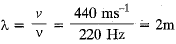Q6. A person is listening to a tone of 500 Hz sitting at a distance of 450 m from the source of the sound. What is the time interval between successive compressions from the source? [Speed of sound in air = 330 ms-1]
Ans: Given: f = 500 Hz, v = 330 ms-1, d = 450 m
Time between two successive compressions is called the time period.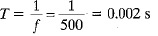Q7. Distinguish between loudness and intensity of sound.
Ans:

 Loudness Intensity 1. Loudness is a measure of the response of our ear to the sound. 1. It is the energy passing per second per unit that are normal to the direction of energy flow. 2. It depends on the intensity as well as the sensitivity of the ear. Therefore, it is not absolute but relative. 2. It does not depend upon the sensitivity of the ear. 3. The unit of loudness is decibel. 3. The unit of intensity is watt/m2.

Page No. 167

Q1. In which of the three media, air, water or iron, does sound travel the fastest at a particular temperature?
Ans: Sound travels fastest in solids due to their high elasticity, so out of given media sound travels fastest in iron.

Page No. 168

Q1. An echo is returned in 3s. What is the distance of the reflecting surface from the source, given that the speed of sound is 342 ms-1?
Ans: If d is the distance of reflecting surface from the source and t is the time interval of echo-return, then
2d = vt ⇒ d = vt/2
Here, v - 342 ms-1, t = 3 s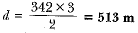Page No. 169

Q1. Why is the ceiling of concert halls curved?
Ans: Ceilings of concert halls are curved so that sound after reflection reaches all corners of the hall.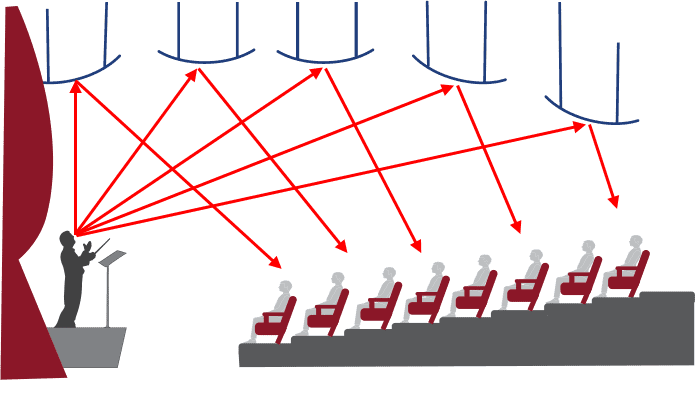Page No. 170

Q1. What is the audible range of the average human ear?
Ans: Audible range for human ear = 20 Hz - 20 kHz

Q2. What is the range of frequencies associated with:
(a) Infrasound
(b) Ultrasound?
Ans: (a) Infrasound: Sound waves between the frequencies 1 to 20-Hz
(b) Ultrasound: Sound waves of frequencies above 20,000 Hz.

Page No. 172

Q1. A submarine emits a sonar pulse, which returns from an underwater cliff in 1.02 s. If the speed of, sound in saltwater is 1531 ms1, how far away is the cliff?
Ans: Given: t = 1.02 s, v = 1531 ms-1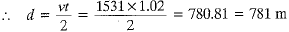Page No. 174

Q1. What is sound and how is it produced?
Ans: A sound is a form of energy that produces the sensation of hearing. It is produced by oscillation/ vibration of particles of a material medium.

Q2. Describe with the help of a diagram, how compressions and rarefactions are produced in the air near a source of the sound.
Ans: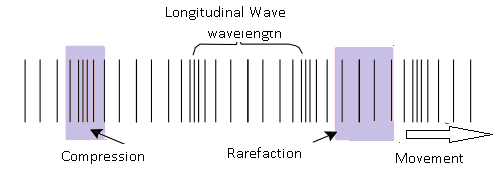Q3. Cite an experiment to show that sound needs a material medium for its propagation.
Ans:
Fix an electric bell inside a jar connected to a vacuum pump. Close the mouth of the jar and ring the bell. Slowly remove the air using the vacuum pump, so that vacuum is created in the jar. The sound will end when all the air is pumped out. This demonstrates the requirement for a medium to carry sound.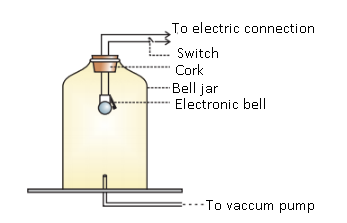Q4. Why is sound wave called a longitudinal wave?
Ans: In sound waves, the vibration of the particles of the medium are along the direction of wave propagation, so sound waves in the air are longitudinal.

Q5. Which characteristic of the sound helps you to identify your friend by his voice while sitting with Miens in a dark room?
Ans: It is the quality of sound (or waveform) that helps us to identify the voice of our friend without seeing him.

Q6. Flash and thunder are produced simultaneously. But thunder is heard a few seconds after the flash is seen, why?
Ans: The speed of light is 3 x 108 m/s while that of sound in air is only 330 m/s.
As Time = Distance/speed
So sound takes more time than light to reach on earth. That is why thunder is heard later than flash is seen.

Q7. A person has a hearing range from 20 Hz to 20 kHz. what are the typical wavelengths of sound waves in air corresponding to these two frequencies? Take the speed of sound in air as 344 ms1.
Ans: For sound waves,

Speed = Wavelength × frequency

v = λ × v

Speed of sound wave in air = 344 m/s

(a) For v = 20 Hz

λ1 = v/v1 = 344/20 = 17.2 m

(b) For v2 = 20,000 Hz

λ2 = v/v2 = 344/20,000 = 0.0172 m

Therefore, for human beings, the hearing wavelength is in the range of 0.0172 m to 17.2 m.

Q8. Two children are at opposite ends of an aluminium rod. One strikes the end of the rod with a stone. Find the ratio of times taken by the sound wave in the air and in aluminium to reach the second child. Given the velocity of sound in air and aluminium are 346 ms1 and 6420 ms1 respectively.
Ans: Consider the length of aluminium rod = d

Speed of sound wave at 25° C, V Al = 6420 ms-1

Time taken to reach other end

T Al = d/ (V Al) = d/6420

Speed of sound in air, V air = 346 ms-1

Time taken by sound to each other end,

T air = d/ (V air) = d/346

Therefore, the ratio of time taken by sound in aluminium and air,

T air / T Al = 6420 / 346 = 18.55

Q9. The frequency of a source of sound is 100 Hz. How many times does it vibrate in a minute?
Ans: Frequency = (Number of oscillations) / Total time

Number of oscillations = Frequency × Total time

Given,

∵ 1 minute = 60 s
Vibrations in 1 s = frequency = 100.
Vibrations in 60 s = 60 x 100 = 6000 times

The source vibrates 6000 times in a minute and produces a frequency of 100 Hz.

Q10. Does sound follow the same laws of reflection as light does? Explain.
Ans: Yes, sound and light follow the same laws of reflection given below :

• Angle of incidence at the point of incidence = Angle of reflection.
• At the point of incidence the incident sound wave, the normal and the reflected sound wave lie in the same plane.

Q11. When a sound is reflected from a distant object, an echo is produced. Let the distance between the reflecting surface and the source of sound production remain the same. Do you hear echo sounds on a hotter day?
Ans: An echo is heard when the time interval between the reflected sound and the original sound is at least 0.1 seconds. As the temperature increases, the speed of sound in a medium also increases. On a hotter day, the time interval between the reflected and original sound will decrease and an echo is audible only if the time interval between the reflected sound and the original sound is greater than 0.1 s.

Q12. Give two practical applications of reflection of sound waves.
Ans: Applications of reflection of sound waves are

• to locate underwater hidden objects such as rocks and icebergs through SONAR.
• to detect any undesired objects in the sky near airports and borders of the country.

Q13. A stone is dropped from the top of a tower 500 m high into a pond of water at the base of the tower. When is the splash heard at the top? Given, g = 10 ms-2 and speed of sound = 340 ms-1.
Ans: Height (s) of tower = 500 m

Velocity (v) of sound = 340 m s−1

Acceleration (g) due to gravity = 10 m s−1

Initial velocity (u) of the stone = 0

Time (t1) taken by the stone to fall to tower base

As per second equation of motion:

s= ut1 + (½) g (t1)2

500 = 0 x t1 + (½) 10 (t1)2

(t1)2 = 100

t= 10 s

Time (t2) taken by sound to reach top from tower base = 500/340 = 1.47 s.

t = t1 + t2

t = 10 + 1.47

t = 11.47 s.

Time for the splash to be heard is 11.47 s from the time the stone is dropped.

Q14. A sound wave travels at a speed of 339 ms-1. If its wavelength is 1.5 cm, what is the frequency of the wave? Will it be audible?
Ans: Given: Velocity of sound, v = 339 ms-1
Wavelength, λ = 1.5 cm - 0.015 m
Frequency, v == 22.6 kHz
This sound is not audible to human beings as frequency is higher than the audible range.

Q15. What is reverberation? How can it be reduced?
Ans: The time from generation of sound until its loudness reduces to zero is called reverberation time. The process due to which the persistence of sound is caused is called reverberation. This is reduced in an auditorium using sound absorbent materials and good absorbers of sound.

Q16. What is the loudness of sound? What factors does it depend on?
Ans: The loudness of sound is a measure of the response of sound to our ears. The loudness of sound is not simply the energy reaching the human ear, but it also tells about the sensitivity of the human ear detecting this energy. The loudness of sound is measured in decibel (dB). As energy reaching the ear depends on the square of the amplitude, so loudness of sound depends on two factors:
(i) Amplitude of sound waves and (ii) Sensitivity of ear.

Q17. Explain how bats use ultrasound to catch their prey.
Ans: The high pitched ultrasonic waves sent by the bats to the prey are received by them as they get reflected from the prey. This makes bats move in the right direction to catch their prey.

Q18. How is ultrasound used for cleaning?
Ans: Ultrasound is used to clean the hard to reach places such as spiral tubes, electronic components etc. Object to be cleaned is placed in the cleaning solution and ultrasonic waves are sent into it. The high frequency of ultrasound detaches the dust, grease and dirt from the object and it gets thoroughly cleaned.

Q19. Explain the working and application of a SONAR.
Ans: SONAR expands into Sound Navigation and Ranging with ultrasonic waves. It is used to locate underwater obstacles or enemy submarines etc. It is used by defence services to maintain track of any trouble due to the enemies from the sea. Sonar works on the principle of finding time taken by ultrasonic sound to travel back to the ship after hitting any object.
It is given by, t = 2d/v where v is the velocity of the wave in the media and d is the distance of the object from the ship. It has a transmitter and receiver to send and receive ultrasonic waves. It is also used to find the depth of the ocean at any point.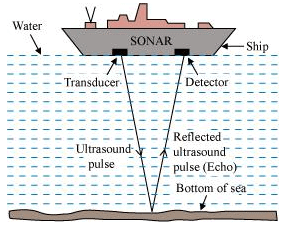Q20. A sonar device on a submarine sends out a signal and receives an echo 5 s later. Calculate the speed of sound in water if the distance of the object from the submarine is 3625 m.
Ans: Given: Time, t = 5s;
Distance between submarine and object
= 3625 m.
Distance travelled by sound
= 3625 m x 2 (sound + echo) = 7250 m
Speed of sound in water =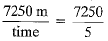= 1450 m/s.

Q21. Explain how defects in a metal block can be detected using ultrasound.
Ans: Ultrasonic waves are allowed to pass through the metal. If the block is flawless, it will pass through it. If there is a crack or deformity, the wave gets reflected. Time taken by the wave to return back is measured and helps to locate the flaw. If the wave is not reflected, it means the metal has no deformity.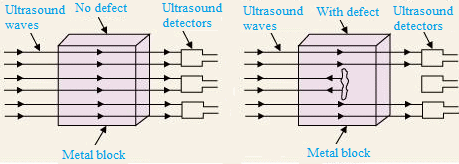Q22. Explain how the human ear works.
Ans: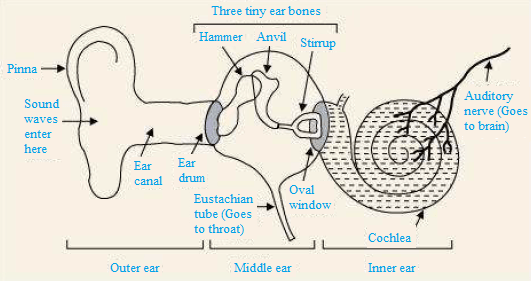Various sounds produced by particles in our surroundings are collected by pinna that transfers these sounds to the eardrum through the ear canal. The eardrum begins to vibrate back and forth briskly as soon as the sound waves fall on it. The vibrating eardrum initiates the small bone hammer to vibrate. These vibrations are passed from the hammer to the third bone stirrup via the second bone anvil. The stirrup strikes the membrane of the oval window to pass its vibration to the cochlea. The liquid in the cochlea produces electrical impulses in the nerve cells. These electrical impulses are carried to the brain by the auditory nerve. They are interpreted by the brain as sound and hence we get a sensation of hearing.

The document NCERT Solutions - Sound Class 9 Notes | EduRev is a part of the Class 9 Course Science Class 9.
All you need of Class 9 at this link: Class 9Use Code STAYHOME200 and get INR 200 additional OFF Use Coupon Code
All Tests, Videos & Notes of Class 9: Class 9

### Top Courses for Class 9## Science Class 9

84 videos|225 docs|163 tests

### Top Courses for Class 9Track your progress, build streaks, highlight & save important lessons and more!

,

,

,

,

,

,

,

,

,

,

,

,

,

,

,

,

,

,

,

,

,

;